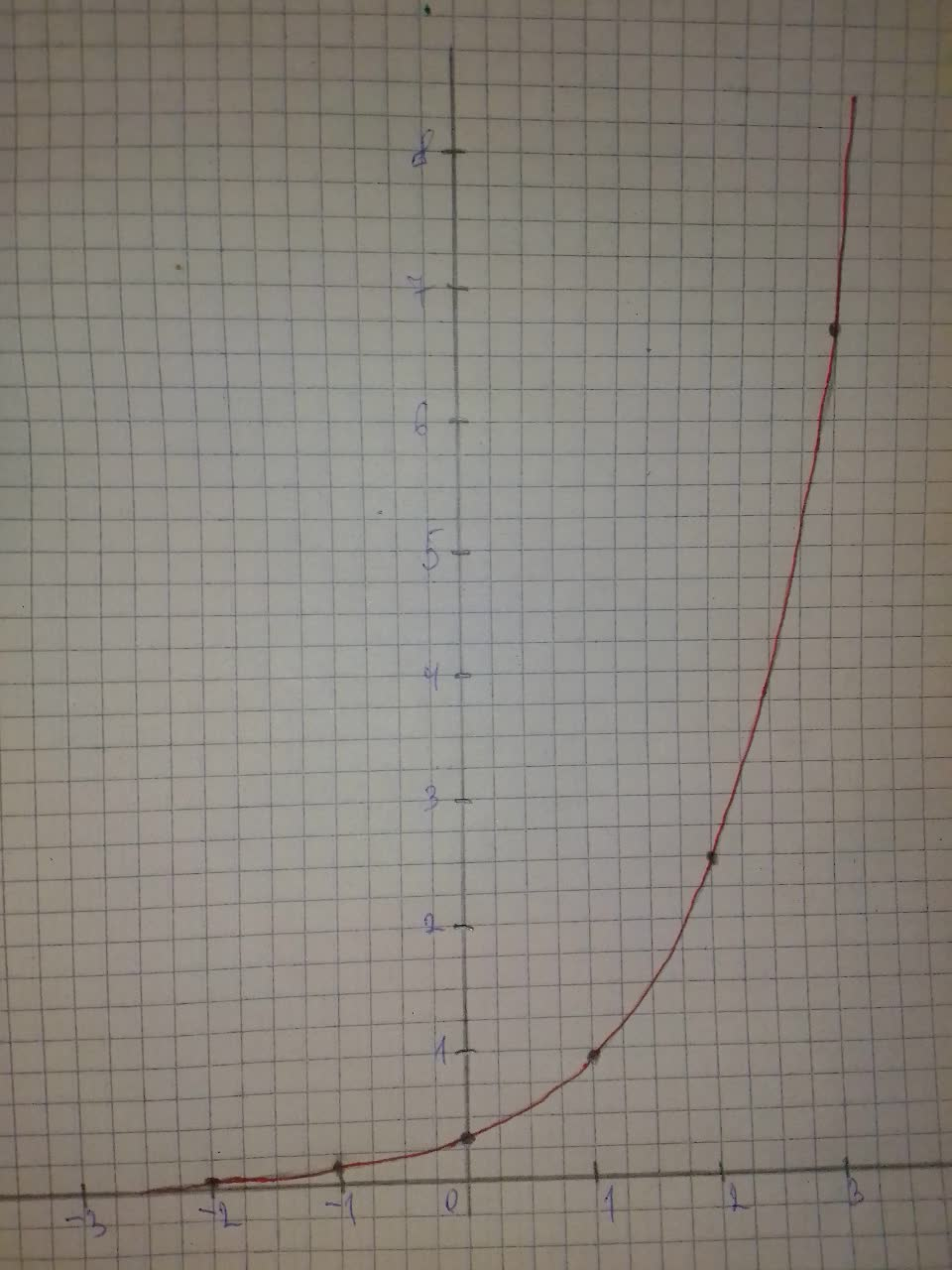Tell whether the function represents exponential growth or exponential decay. Then graph the function f(x)=\frac{1}{3}e^{x}Burhan Hopper 2021-07-28 Answered
Tell whether the function represents exponential growth or exponential decay. Then graph the function.
$$\displaystyle{f{{\left({x}\right)}}}={\frac{{{1}}}{{{3}}}}{e}^{{{x}}}$$

Want to know more about Exponential growth and decay?

• Questions are typically answered in as fast as 30 minutes

Solve your problem for the price of one coffee

• Math expert for every subject
• Pay only if we can solve itTheodore Schwartz

Step 1
$$\displaystyle{f{{\left({x}\right)}}}={\frac{{{1}}}{{{3}}}}{e}^{{{x}}}$$
Step 2
We identify the value of the base. The base, e, is greater than 1, thus the function represents exponential growth.
Step 3
$$\begin{array}{|c|c|}\hline x & y \\ \hline -2 & 0.045 \\ \hline -1 & 0.123\\ \hline 0 & 0.333\\ \hline 1 & 0.906\\ \hline 2 & 2.463\\ \hline 3 & 6.695\\ \hline \end{array}$$
Step 4
We plot the points from the table. Then we draw a smoth curve from left to right, that begins just above the x-axis, passes through the plotted points and moves up to the right.